Polynomial and polynomial matrix glossary

Polynomial matrices

Index | Top

We review some definitions and basic facts related to polynomial matrices.

A k×m polynomial matrix is a matrix of the form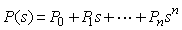where s is an indefinite variable (usually taking its values in the complex plane), and the k×m constant matrices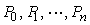coefficient matrices. Usually, unless stated otherwise, we deal with real polynomial matrices, whose coefficient matrices are real.

If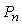is not the zero matrix then we say that P has degree n. Ifis the unit matrix then P is said to be monic.

Tall and wide A polynomial or other matrix is tall if it has at least as many rows as columns. It is wide if it has at least as many columns as rows.
Rank

Index | Top

A polynomial matrix P has full column rank (or full normal column rank) if it has full column rank everywhere in the complex plane except at a finite number of points. Similar definitions hold for full row rank and full rank.

The normal rank of a polynomial matrix P equals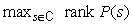Similar definitions apply to the notions of normal column rank and normal row rank.

A square polynomial matrix is nonsingular if it has full normal rank.

Row and column degrees

Index | Top

Let the elements of the k×m polynomial matrix P be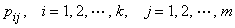Then the numbers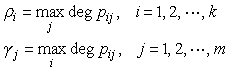are the row and the column degrees of P, respectively.

Index | Top

Suppose that P has column and row degrees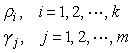respectively.

The column leading coefficient matrix of P is the constant matrix whose (i, j) entry is the coefficient of the term with power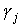of the (i, j) entry of P.

The row leading coefficient matrix of P is the constant matrix whose (i, j) entry is the coefficient of the term with power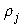of the (i, j) entry of P.

Column and row reduced A polynomial matrix is column reduced if its column leading coefficient matrix has full column rank. It is row reduced if its row leading coefficient matrix has full row rank.
Conjugate

Index | Top

If P is a polynomial matrix then its conjugate P* is the polynomial matrix defined by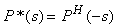The superscript H indicates the complex conjugate transpose.

Para-Hermitian A square polynomial matrix P is para-Hermitian if P* = P.
Diagonally reduced

Index | Top

The m×m para-Hermitian polynomial matrix P is diagonally reduced if there exist half diagonal degrees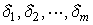so that the diagonal leading coefficient matrix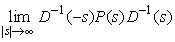exists and is nonsingular. D is the diagonal polynomial matrix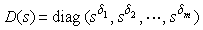Roots

Index | Top

The roots or zeros of a polynomial matrix P are those points in the complex plane where P loses rank.

If P is square then its roots are the roots of its determinant det P, including multiplicity.

Primeness A polynomial matrix P is left prime if it has full row rank everywhere in the complex plane. It is right prime if it has full column rank everywhere in the complex plane.
Coprimeness

Index | Top

The N polynomial matrices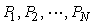with the same numbers of rows are left coprime if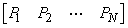is left prime. If the N polynomila matrices all have the same numbers of columns then they are right coprime if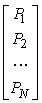is right prime.

Unimodular A square polynomial matrix U is unimodular if its determinant det U is a nonzero constant. The inverse of a unimodular polynomial matrix is again a polynomial matrix.
Matrix pencil

Index | Top

Matrix pencils are matrix polynomials of degree 1, such as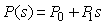Matrix pencils are often represented as polynomial matrices of the special form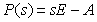but we shall normally consider matrix pencils as general polynomial matrices of degree 1.

Elementary row and column operations

Index | Top

There are three basic elementary row operations:
• multiplying a row by a nonzero constant, such as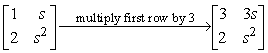• interchanging two rows, such as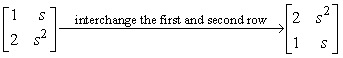• adding a polynomial multiple of one row to another, such as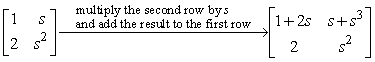Elementary column operations are defined analogously.

Diophantine equations

Index | Top

The simplest type of linear scalar polynomial equation - called Diophantine equation after by the Alexandrian mathematician Diophantos (A.D. 275) is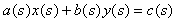The polynomials polynomials a, b and c are given while the polynomials x and y are unknown. The equation is solvable if and only if the greatest common divisor of a and b divides c. This implies that with relatively a and b coprime the equation is solvable for any right hand side polynomial, including c = 1.

The Diophantine equation possesses infinitely many solutions whenever it is solvable. If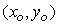is any (particular) solution, then the general solution is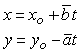where t is an arbitrary polynomial (the parameter) and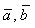are coprime polynomials such that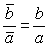If the a and b themselves are coprime then one can naturally take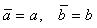Among all the solutions of Diophantine equation there exists a unique solution pair (x, y) characterized by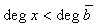There is another (generally different) solution pair characterized by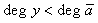The two solution pairs coincide only if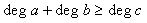Bézout equations

Index | Top

A Diophantine equation with 1 on its right hand side is called a Bézout equation. It may look like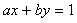with a and b given polynomials and x and y unknown.

Zeroing

Index | Top

Theoretically, the degree of a polynomial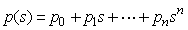is n whenever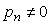. In numerical computations, however, one often encounters the case of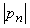very small (much smaller than the other coefficients) yet non-zero.

By way of example, consider two simple polynomials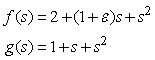where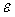is almost (but not quit) zero. When computing the difference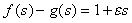a question on its degree may arise. It is necessary to compare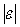with the norms of the other coefficients to decide whether or not the corresponding term should be completely deleted. This process is called zeroing. The performance of many algorithms for polynomial problems critically depends on the way zeroing is done, in particular when elementary operations are used.

Sylvester resultant matrix

Index | Top

The Sylvester resultant matrix corresponding to the polynomials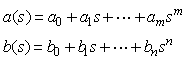is the (m+n)×(m+n) constant matrix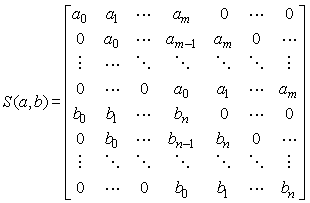The resultant matrix is nonsingular if and only if the polynomials a and b are coprime.

Divisors and multiples

Index | Top

Consider polynomials a, b and c such that a = bc. We say that b is a divisor of a of or a is a multiple of b, and write b|a. This is sometimes also stated as b divides a.

If a polynomial g divides both a and b then g is called a common divisor of a and b. If, furthermore, g is a multiple of every common divisor of a and b then g is a greatest common divisor of a and b. If the only common divisors of a and b are constants then the polynomials a and b are coprime.

If a polynomial m is a multiple of both a and b then m is called a common multiple of a and b. If, furthermore, m is a divisor of every common multiple of a and b then it is a least common multiple of a and b.

Next consider now polynomial matrices A, B and C of compatible sizes such that A = BC. We say that B is a left divisor of A or A is a right multiple of B.

If a polynomial matrix G is a left divisor of both A and B then G is called a common left divisor of A and B. If, furthermore, G is a right multiple of every common left divisor of A and B then it is a greatest common left divisor of A and B. If the only common left divisors of A and B are unimodular matrices then the polynomial matrices A and B are left coprime.

If a polynomial matrix M is a right multiple of both A and B then M is called a common right multiple of A and B. If, furthermore, L is a left divisor of every common
right multiple of A and B then G is a least common right multiple of A and B.

Right divisors, left multiples, common right divisors, greatest common right divisors, common left multiples, and least common left multiples are similarly defined.

 Index Bézout equations coefficient matrix column degrees column leading coefficient matrix column rank column reduced conjugate coprime degree diagonal leading coefficient matrix diagonally reduced Diophantine equations divisor elementary row and column operations full normal rank full rank half diagonal degrees left coprime row reduced left prime matrix pencil monic multiple nonsingular normal rank para-Hermitian prime rank right coprime roots row degrees row leading coefficient matrix row rank Sylvester resultant matrix tall unimodular zeroing Top